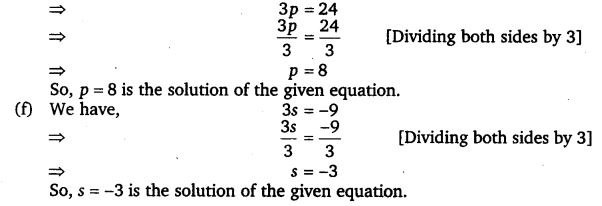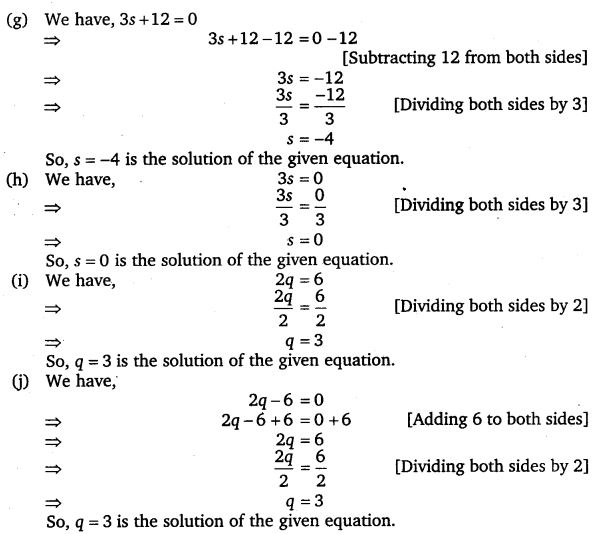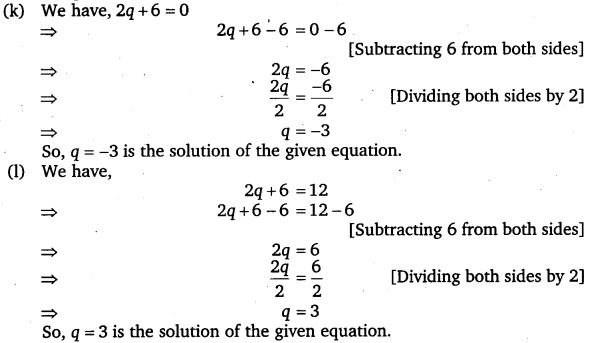# Class 7 Maths NCERT Solutions Chapter – 4 Simple Equations EX – 4.2

## Simple Equations

Question 1.
Give first the step you will use to separate the variable and then solve the equation :
(a)
x – 1 = 0
(b) x + 1= 0
(c) x – 1 = 5
(d) x + 6 = 2
(e) y – 4 = -7
(f) y – 4 = 4
(g) y + 4 = 4
(h) y – 4 = -4

Solution:
(a) We have, x – 1 = 0
In order to solve this equation, we have to get x by itself on the L.H.S. To get x by itself on the L.H.S., we need to shift -1. This can be done by adding 1 to both sides of the given equation.
x – 1 + 1 = 0 + 1 [ Adding 1 to both sides ]
x = 1 [ ∵ -1 + 1 = 0 and 0 + 1 = 1 ]

(b) We have, x + 1 = 0
In order to get x by itself on the L.H.S., we need to shift 1. This can be done by subtracting 1 from both sides of the given equation.
x + 1 – 1 = 0 – 1 [Subtracting 1 from both sides]
⇒ x = -1 [∵ 1 – 1 = 0, 0 – 1 = -1 ]
So, x = -1 is the solution of the given equation.

(c) We have, x – 1 = 5
In order to solve this equation, we have to get x by itself on the L.H.S. To get x by itself on the L.H.S., we need to shift -1. This can be done by adding 1 to both sides of the given equation.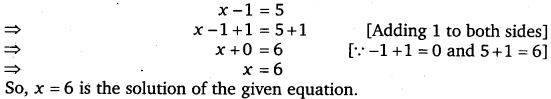(d) We have, x + 6 = 2
In order to solve this equation, we have to get x by itself on the L.H.S. To get x by itself on the L.H.S., we need to shift 6. This can be done by subtracting 6 from both sides of the given equation.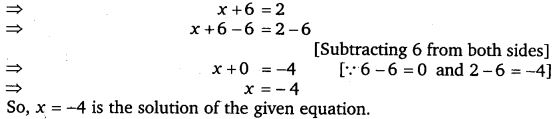(e) We have, y – 4 = -7
In order to solve this equation, we have to get y by itself on the L.H.S. To get y by itself on the L.H.S.,we need to shift -4. This can be done by adding 4 to both sides of the given equation.(f) We have, y – 4 = 4
In order to solve this equation, we have to get y by itself on the L.H.S. To get y by itself on the L.H.S., we need to shift -4. This can be done by adding 4 to both sides of the given equation.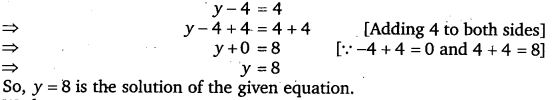(g) We have, y + 4 = 4
In order to solve this equation, we have to get y by itself on the L.H.S. To get y by itself on the L.H.S., we need to shift 4. This can be done by subtracting 4 from both sides of the given equation.(h) We have, y + 4 = -4
In order to solve this equation, we have to get y by itself on the L.H.S. To get y by itself on the L.H.S., we need to shift 4. This can be done by subtracting 4 from both sides of the given equation.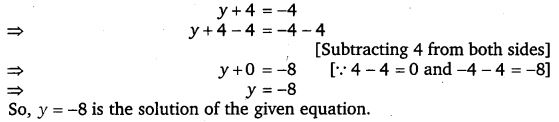Question 2.
Give first the step you will use to separate the variable and then solve the equation :
(a)
3l = 42
(b)$\frac { b }{ 2 }$ = 6
(c)$\frac { p }{ 7 }$ = 4
(d) 4x = 25
(e) 8y = 36
(f)$\frac { z }{ 3 }$ =$\frac { 5 }{ 4 }$
(g)$\frac { a }{ 5 }$ =$\frac { 7 }{ 15 }$
(h) 20t = -10

Solution:
(a) We have, 3l = 42
In order to solve this equation, we have to get l by itself on the L.H.S. For this, 3 has to be removed from the L.H.S. This can be done by dividing both sides of the equation by 3.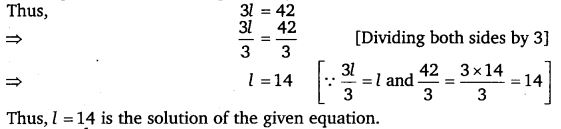(b) We have,$\frac { b }{ 2 }$ = 6
In order to solve this equation, we have to get b by itself on the L.H.S. To get b by itself on L.H.S., we have to remove 2 from L.H.S. This can be done by multiplying both sides of the equation by 2. Thus, we have(c) We have,$\frac { p }{ 7 }$ = 4
In order to solve this equation, we have to get p by itself on the L.H.S. To get p by itself on L.H.S., we have to remove 7 from L.H.S. This can be done by multiplying both sides of the equation by 7. Thus, we have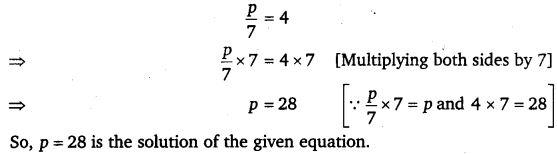(d) We have, 4x = 25
In order to solve this equation, we have to get x by itself on the L.H.S. For this, 4 has to be removed from the L.H.S. This can be done by dividing both sides of the equation by 4.(e) We have, 8y = 36
In order to solve this equation, we have to get y by itself on the L.H.S. For this, 8 has to be removed from the L.H.S. This can be done by dividing both sides of the equation by 8.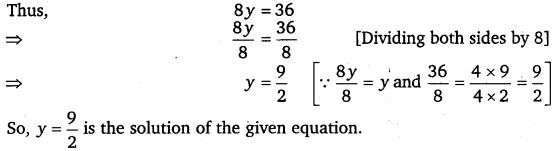(f) We have,$\frac { z }{ 3 }$ =$\frac { 5 }{ 4 }$
In order to solve this equation,we have to get z by itself on the L.H.S. To get z by itself on L.H.S., we have to remove 3 from L.H.S. This can be done by multiplying both sides of the equation by 3.
Thus, we have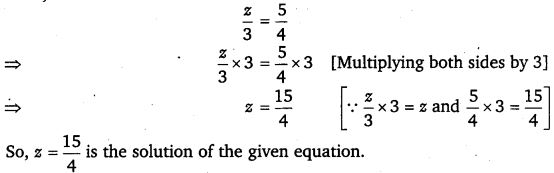(g) We have,$\frac { a }{ 5 }$ =$\frac { 7 }{ 15 }$
In order to solve this equation, we have to get a by itself on the L.H.S. To get a by itself on L.H.S., we have to remove 5 from L.H.S. This can be done by multiplying both sides of the equation by 5.(h) We have, 20t = -10
In order to solve this equation, we have to get t by itself on the L.H.S. For this, 20 has to be removed from the L.H.S. This can be done by dividing both sides of the equation by 20.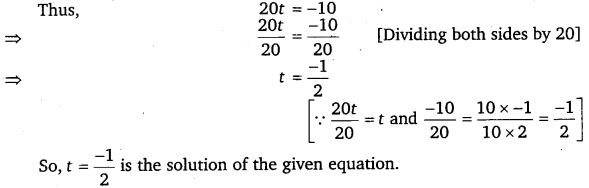Question 3.
Give the steps you will use to separate the variable and then solve the equation :
(a)
3n – 2 = 46
(b) 5m + 7 = 17
(c)$\frac { 20p }{ 3 }$ = 40
(d)$\frac { 3p }{ 10 }$ = 6

Solution:

Question 4.
Solve the following equations :
(a)
10p = 100
(b) 10p + 10 = 100
(c)$\frac { p }{ 4 }$ = 5
(d)$\frac { -p }{ 3 }$ = 5
(e)$\frac { 3p }{ 4 }$ = 6
(f) 3s = -9
(g) 3s + 12 = 0
(h) 3s = 0
(i) 2q = 6
(j) 2q – 6 = 0
(k) 2q + 6 = 0
(l) 2q + 6 = 12

Solution: